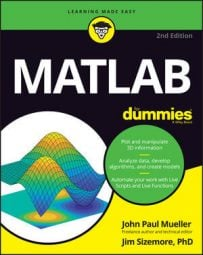##### MATLAB For DummiesThe MATLAB syntax is a set of rules that you use to tell MATLAB what to do. It’s akin to learning another human language, except that the MATLAB syntax is significantly simpler than any human language. In order to communicate with MATLAB, you must understand its language, which is essentially a form of math. Because you already know math rules, you already know many MATLAB rules as well.

## Adding, subtracting, multiplying, and dividing

MATLAB is a math-based language, so it pays to review the basic rules for telling MATLAB how to perform basic math tasks. Of course, MATLAB performs the basic math functions:

• + or plus(): Adds two numbers. For example, you can use 3 + 4 or plus(3, 4) to obtain a result of 7.

• - or minus(): Subtracts two numbers. For example, you can use 3 - 4 or minus(3, 4) to obtain a result of –1.

• * or times(): Multiplies two numbers. For example, you can use 3 * 4 or times(3, 4) to obtain a result of 12.

• / or rdivide(): Performs right division, which is the form of division you likely learned in school. For example, you can use 3 / 4 or rdivide(3, 4) to obtain a result of 0.75.

• or ldivide(): Performs left division, which is also called “goes into” or, as you learned in third grade, “guzintas.” You know (say this out loud), 5 “guzinta” 5 once, 5 “guzinta” 10 twice, 5 “guzinta” 15 three times, and so on. For example, you can use 3 4 or ldivide(3, 4) to obtain a result of 1.3333.

Most MATLAB operators are binary, which means that they work on two values. For example, 3 + 4 has two values: 3 and 4. However, some operators are unary, which means that they work on just one value. Here are the basic unary operators:

• + or uplus(): Returns the unmodified content of a value or variable. For example, +1 or uplus(1) is still equal to 1.

• - or uminus(): Returns the negated content of a value or variable. For example, -1 or uminus(1) returns –1. However, -–1 or uminus(–1) returns 1 (the negative of a negative is a positive).

In some cases, you don’t want a floating-point result from division. To perform integer division, you have to use special functions — you can’t just use operators for the simple reason that no operators are associated with these math tasks. Here are the functions associated with integer math:

• idivide(): Performs integer division. You supply two values or variables as input, along with an optional modifier that tells MATLAB how to perform rounding.

To use the idivide() function, you must specify that the input values are integers. For example, idivide(int32(5), int32(3)) provides an output of 1. Here is a list of the modifiers you use to provide different rounding effects:

• ceil: Rounds toward positive infinity. For example, idivide(int32(5), int32(3), ‘ceil’) produces an output of 2 and idivide(int32(5), int32(–3), ‘ceil’) produces an output of –1.

• fix: Rounds toward zero. For example, idivide(int32(5), int32(3), ‘fix’) produces an output of 1 and idivide(int32(5), int32(–3), ‘fix’) produces an output of –1.

• floor: Rounds toward negative infinity. For example, idivide(int32(5), int32(3), ‘floor’) produces an output of 1 and idivide(int32(5), int32(–3), ‘floor’) produces a result of –2.

• round: Rounds to the nearest integer. For example, idivide(int32(5), int32(3), ‘round’) produces an output of 2 and idivide(int32(5), int32(–3), ‘round’) produces an output of –2.

• mod(): Obtains the modulus after division. For example, mod(5, 3) produces an output of 2 and mod(5, –3) produces an output of –1.

• rem(): Obtains the remainder after division. For example, rem(5, 3) produces an output of 2 and rem(5, –3) produces an output of –2.

Rounding can be an important feature of an application because it determines the approximate values the user sees. You can round any formula that you want to produce an integer output. Here are the rounding functions:

• ceil(): Rounds toward positive infinity. For example, ceil(5 / 3) produces an output of 2 and ceil(5 / –3) produces an output of –1.

• fix(): Rounds toward zero. For example, fix(5 / 3) produces an output of 1 and fix(5 / –3) produces an output of –1.

• floor(): Rounds toward negative infinity. For example, floor(5 / 3) produces an output of 1 and floor(5 / –3) produces an output of –2.

• round(): Rounds toward nearest integer. For example, round(5 / 3) produces an output of 2 and round(5 / –3) produces an output of –2.

## Working with exponents

You use the caret (^) to raise a number to a particular power. MATLAB can handle negative, fractional, and complex number bases as exponents. Here are some examples of exponents:

• 10^3 = 1000

• 2^10 = 1024

• 2.5^2.5 = 9.8821

• 2^-4 = 0.0625

• 2^I = 0.7692 + 0.6390i

• i^I = 0.2079